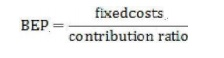Home | Important Questions and Answers: Cost And Break Even Analyses

# Important Questions and Answers: Cost And Break Even Analyses

Civil - Engineering Economics and Cost analysis - Cost And Break Even Analyses

COST AND BREAK EVEN ANALYSIS

1.       What are the uncertainties a firm faces?

1)      Dynamic nature of consumer needs

2)      Diverse nature of competition

3)      Uncontrollable nature of most elements of cost

4)      Continuous technological developments

2.       How is cost-volume-profit relationship determined?

The most important method of determining cost-volume-profit relationship is Break

even Analysis.

3.       What is Break even Analysis?

The method of determining the cost-volume-profit relationship is known as Break even

Analysis.

4.       Who are benefitted through Break even Analysis?

Break even Analysis is useful for business executives, but also for an entrepreneur who is

on the threshold of setting up his own unit.

5.       What is the usefulness Break even Analysis?

Break even Analysis is valuable for project appraisal executives, business

students, accountants etc.

6.       How is the knowledge of Break even Analysis is helpful to business consultant?

The knowledge of Break even Analysis is helpful to business consultant is useful in order

to provide right recommendations to their clients.

7.       What does break even Analysis involves?

Break even Analysis the study of revenue and costs of a firm in relation to its volume of sales and specifically the determination of that volume at which the firms costs and revenue will be equal.

8. What is breakeven point?

Breakeven point is defined as that level of sales at which total revenue is equal to total costs and the net income is equal to zero.

9. Write the relationship between breakeven point and variable cost?12.            How is BEP determined?

BEP's are determined as

In terms of physical units In terms of money

13. What is break even chart?

Break even chart is defined as 'a graphical presentation of fixed costs, variable costs and sales revenue for various volumes of operations. It illustrates the profits or losses incurred at different volumes of operations, the breakeven point and margin of safety'.

14.Give the formula for Selected operating point.15.            Define (P/V) Ratio.

It is the ratio of contribution to sales, which is expressed in terms of percentages. It is also called as 'Contribution Ratio'.

16. How is Contribution Margin per unit Determined? Contribution Margin=Sales-Variable costs

17. Define Marginal Ratio.

It is the ratio of contribution to sales, which is expressed in terms of percentages. It is also called as 'Contribution Ratio'.

20. What are the uses of BEA?

It predicts the effects of change in price on sales.

It predicts the effects of change on profitability of changes in costs and efficiency. 21. Write down the limitations of break even Analysis?

BEP Analysis assumes costs and revenue to be linear in function. This practice is not true. Break Even Chart is useful only for single product companies.

22.            How is BEP determined In terms of physical units?23.            How is BEP determined In terms of money?24.            How is Contribution Ratio Determined?Study Material, Lecturing Notes, Assignment, Reference, Wiki description explanation, brief detail
Civil : Engineering Economics and Cost analysis : Cost And Break Even Analyses : Important Questions and Answers: Cost And Break Even Analyses |